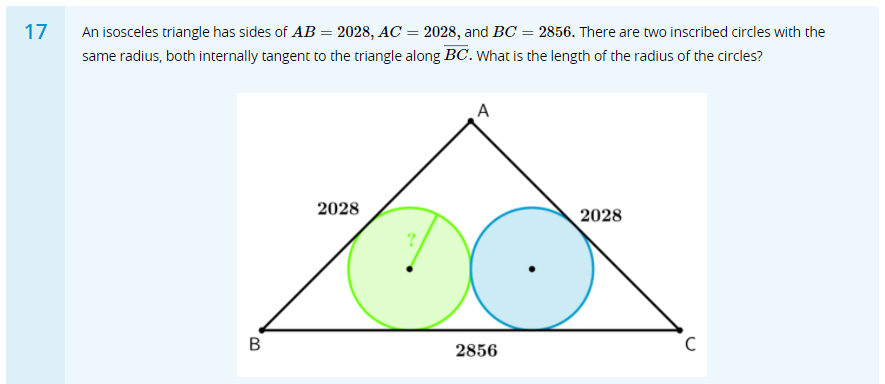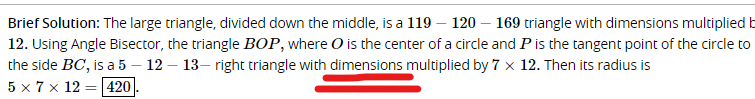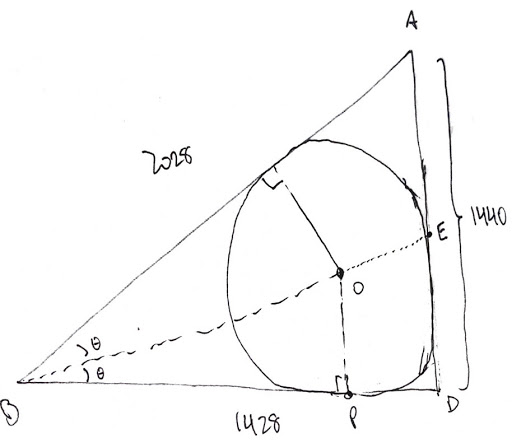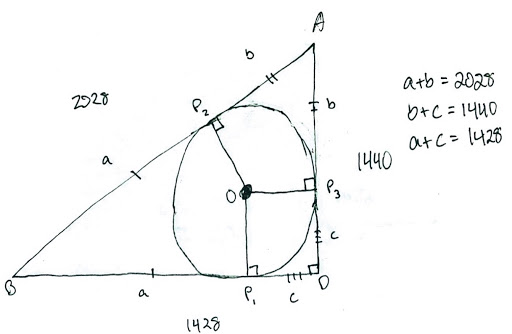# q's from M2 final @17

• Hey, y'all again!

I have attached a question that comes from the M2 final challenge. Can anyone explain to me what "dimension" means in the brief solution?• @victorioussheep Dimensions just means the measurements. In the problem, it's saying that since all the measurements of the right triangle are multiplied by $$7 \cdot 12$$, then all the measurements of the circle must be multiplied by the same thing tooThat's where 3D (3-dimensional) objects get their name. It's because they have three dimensions (measurements): length, width, and height.

2D (2-dimensional) objects, which are anything completely flat, only have two dimensions: length and width.

That must mean that 1D objects (1-dimensional) only have length! Can you think of anything that might be 1D?

$$\text{}$$

If you guessed a line, then you're absolutely correct• @quacker88 why does 5 needs to multiply the dimension? I thought 5 is just the length of the triangle(or the base).

• @victorioussheep Here's a diagram that I drew:(only drew half of it because that's all we need)
So, first off, the length of $$\overline{AD}$$ comes from the Pythagorean Theorem. Or, by chance, if you happen to remember that $$119-120-169$$ is a Pythagorean triple, $$1428-1440-2028$$ is exactly the same triangle, except all the dimensions are multiplied by $$12$$. What the problem states is that $$\triangle BOP$$ happens to be similar to a $$5-12-13$$ triangle. The solution took a huge shortcut, but the idea is that you'll eventually find out that the dimensions of $$\triangle BOP$$ are $$420-1008-1092$$, which is exactly $$84$$ times the dimensions of a $$5-12-13$$ triangle. I know that the solution says to use angle bisector, but I can't think of a way to do it that isn't tedious, maybe the other mods could weigh in on that.

Here's how I did it though-- as long as you get that $$\overline{AD}$$ is $$1440$$, recall that two tangents of a circle that meet have the same length.
Let's redraw the diagram:So, $$\overline{BP_1} \cong \overline{BP_2}, \overline{AP_2} \cong \overline{AP_3}, \overline{DP_1} \cong \overline{DP_3},$$ and I labeled them $$a, b, c$$.
If you want to prove them, here's how:
To prove that $$\overline{BP_1} \cong \overline{BP_2}$$, draw line $$\overline{BO}$$ and prove that triangles $$\triangle{BOP_1}$$ and $$\triangle{BOP_2}$$ are congruent (hint: they're both right triangles!).
You can do the same with all of the other lines.

Anyways, knowing this, we can set up a system of equations, like the one I did to the right of the triangle. Then, notice that the radius of the circle is equivalent to $$c$$ because quadrilateral $$OP_1DP_3$$ is a square. Solving for $$c$$ should give you $$\boxed{420}$$.

Hope this makes sense! I know that it isn't the method that the solution used, but hopefully at least the part about multiplying the dimensions is clearer.
Also, if the other mods want to weigh in on the angle bisector method, that'd be great